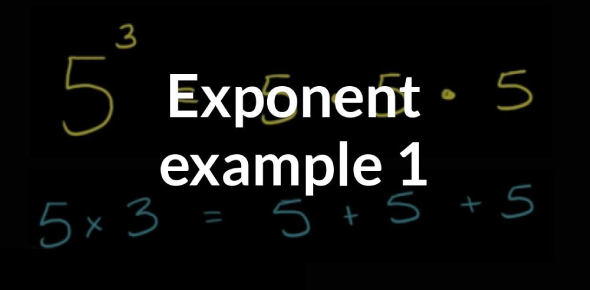# A Practice Quiz On Exponent! Math Trivia Test

15 Questions | Total Attempts: 112SettingsWould you like to take a practice quiz on exponents? Can you ACE this quiz? An exponent is a number that tells us to multiply the same number by itself for a specified number of times. An example of how to utilize exponents in mathematics is to multiply the number zero times. This procedure guarantees that the answer will always be zero. Take this quiz and see how much you know about exponents.

• 1.
When multiplying similar terms, the exponents are
• 2.
When dividing similar terms, the divisor exponents are
• A.

• B.

Subtracted

• C.

Multiplied

• 3.
If an expression is contained inside brackets, and the entire expression is raised to a power, the expression's exponents are added.
• A.

True

• B.

False

• 4.
A3 x a2
• A.

A^6

• B.

A^1

• C.

A^5

• 5.
Ac4 x a3c2
• A.

A^4c^6

• B.

A^3c^6

• C.

A^3c^8

• 6.
4a3 x 3a5
• A.

7a^8

• B.

7a^15

• C.

12a^15

• D.

12a^8

• 7.
A5a3  =
• 8.
A7c3a7c   =
• 9.
A3a5  =
• A.

1/a^2

• B.

A^2

• C.

A^8

• 10.
21a37a4   =
• A.

3a

• B.

1/3a

• C.

3/a

• D.

1/a^7

• 11.
4a5c316a3c4  =
• A.

A^2c/4

• B.

A^2/4c

• C.

1/4a^2c

• 12.
(a4)6
• A.

A^24

• B.

A^46

• C.

A^10

• D.

A^2

• 13.
(3a4)2
• 14.
(2a3c5)3
• A.

6a^9c^125

• B.

8a^6c^8

• C.

6a^27c^125

• D.

8a^9c^15

• 15.
(4a3c2/a4c2)2
Related TopicsBack to top